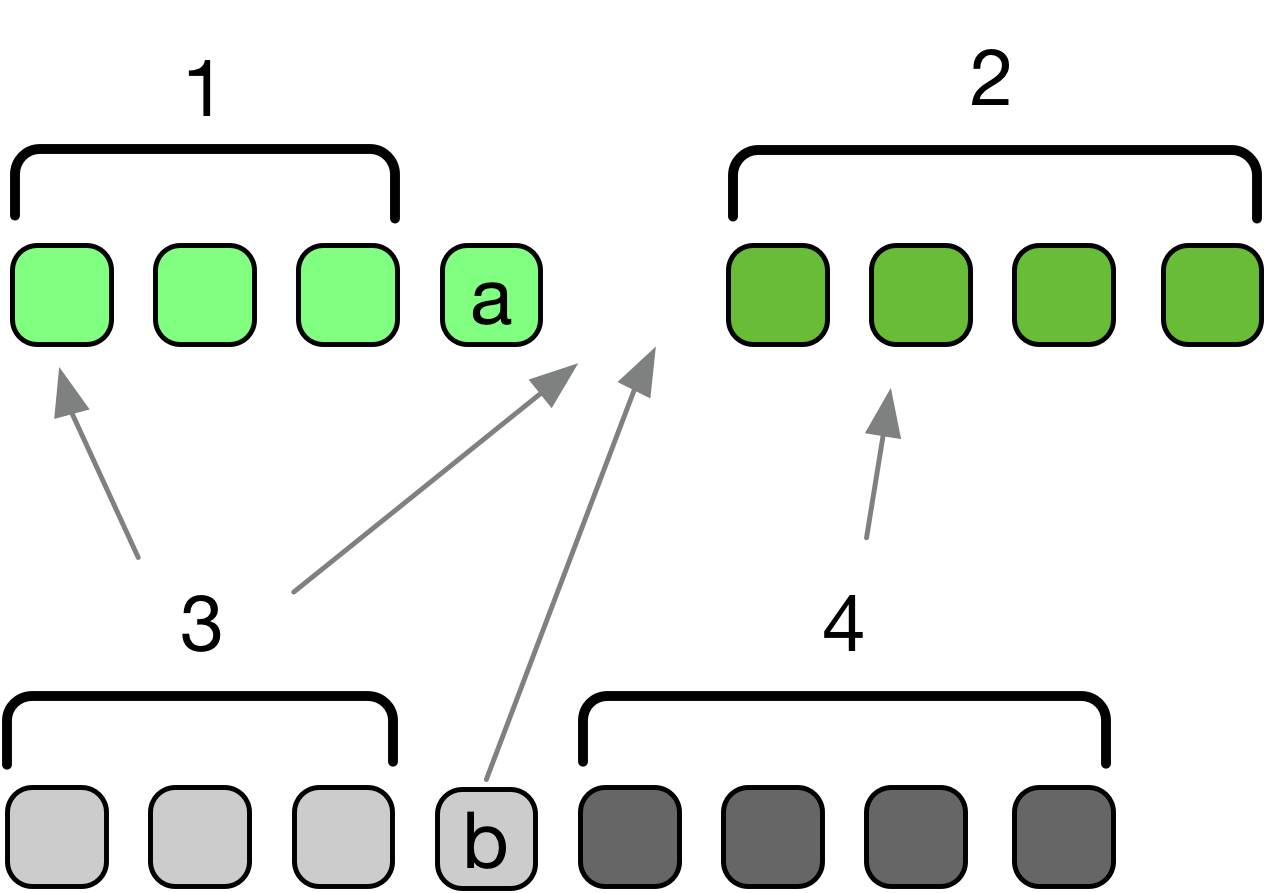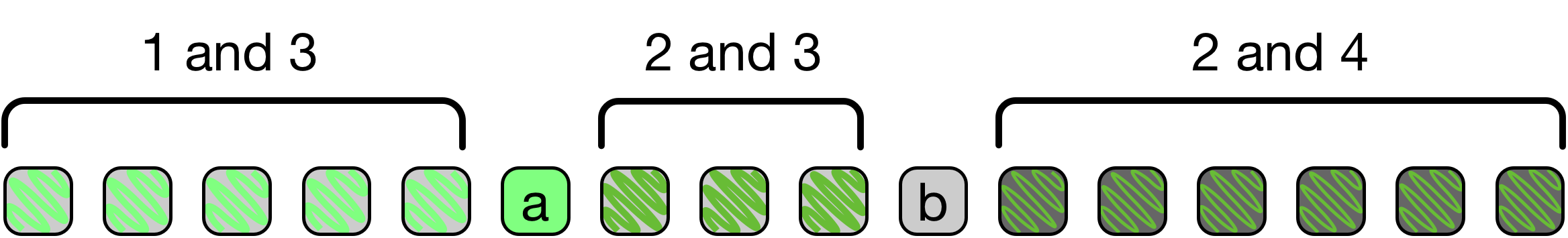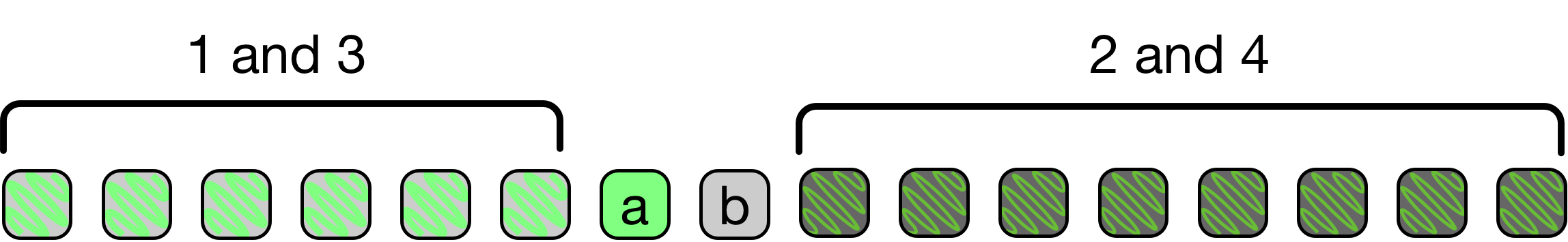# Top Interview Questions and Tricks #2 - Median of Two Sorted Arrays and Divide and Conquer

Median of two sorted arrays is a great problem to do when preparing for interviews.

## Why it’s important

• It’s asked a lot - there a huge number of leetcode upvotes (1800) and submissions (> 1.5 million).
• People find it pretty difficult compared to most interview questions - there seems to be a lot of wrong, O(n log n) or O(n) ‘solutions’ floating around even though the question asks for a O(log n) solution.
• It’s a good example of a divide and conquer algorithm, a common solution technique that comes up a lot in interviews.

## The Problem - (From Leetcode) Median of Two Sorted Arrays

There are two sorted arrays nums1 and nums2 of size m and n respectively.

Find the median of the two sorted arrays. The overall run time complexity should be O(log (m+n)).

Example 1:

nums1 = [1, 3] nums2 = 

The median is 2.0

Example 2:

nums1 = [1, 2] nums2 = [3, 4]

The median is (2 + 3)/2 = 2.5

## The Solution

### Brute force Solutions (O(n log n) and O(n))

If we had a single sorted array, the median would simply be the middle element (the n/2nd element in the odd case and the average of the elements at index n/2 - 1 and n/2 in the even case).

The simplest brute force solution is to simply append one array to the other, sort, and grab the middle element(s). In python3 this looks like (// is integer divide in python3):

def findMedianSortedArrays(self, array1, array2):
array1.extend(array2)
array1.sort()
n = len(array1)
if n % 2 == 0:
return (array1[n // 2] + array1[n // 2 - 1]) / 2
else:
return array1[n // 2]


This actually passes the leetcode test cases but will not be accepted in a big 4 tech interview. It doesn’t even take advantage of the fact that the arrays were sorted!

A better, but still unacceptable, solution (that is linear instead of $O((n+m) \space log (n+m))$, is to simply merge (similar to the merge in mergesort) until we reach the needed element(s).

In C++:

int findKth(int k, vector<int>& A, vector<int>& B) {
// We use i to indicate our position in A and j to indicate our position in B.
int i = 0; int j = 0;
for (; k > 0; k--) {
// We handle the case where we've used all elements from one of the
// arrays first and then skip past the smallest element.
if (i >= A.size()) return B[k];
else if (j >= B.size()) return A[k];
else if (A[i] < B[j]) ++i;
else ++j;
}
if (i >= A.size()) return B[j];
if (j >= B.size()) return A[i];
return A[i] < B[j] ? A[i] : B[j];
}

double findMedianSortedArrays(vector<int>& A, vector<int>& B) {
int t = A.size() + B.size();
if (t % 2 == 1) return findKth(t/2, A, B);
return (findKth(t/2 - 1, A, B) + findKth(t/2, A, B))/2.0;
}


The question asks for a $O(log(n + m))$ solution so let’s try to find something better.

### The Divide and Conquer Solution

Since we’re looking for a $O(log(n+m))$ solution, we have a strong hint that divide and conquer should work.

Looking at our linear solution, we can see that if we could get the k-th item in $O(log(n))$ time, instead of $O(n)$ time, we’ll have a $O(log(n + m))$ solution.

Let’s try to get the k-th value by:

1. Defining a range that definitely contains the kth item.
2. Repeatedly eliminating half (or any fraction) of the elements in that range.

#### 1. Getting the initial range

Any elements with index > k in either array are definitely too big to be the k-th element in the joined array. So, for our initial array we can consider:

• Array1: [0, k]
• Array2: [0, k]

#### 2. Eliminating Possibilities

Can we repeatedly eliminate a fraction of the possibilities?

To define the initial range, we eliminated values that were too big to be the k-th value. Can we prove there are values that are too small to be the k-th value?

Since we have two arrays and we’re hoping to eliminate k/2 possiblities (and using binary search as inspiration), let’s divide at the k/2nd value in each array and see if we can prove some values are too small.1

We’ll call the value at index k/2 in Array1, a, and the value at k/2 in Array2, b.

Then, we have 4 sections:Suppose that a <= b (Without loss of generality2) , how many values are bigger than the elements in section 1 (including a)?

Let’s imagine we merge array B into array A. Where could elements of array B go?If we think through where elements of each section would end up, we’ll end up imagining something like this:Now, let’s imagine the scenario where a is as big as possible, it will look something like:What’s the largest index that a can have?

Section 3 has k/2 - 1 elements and Section 1 has k/2 - 1 elements, so a is at most the k - 1st element3. Since (k/2 - 1 + k/2 - 1 + 1 = k - 1)

So, if eliminate elements in section 1 (and a itself), we’ll be able to create a smaller instance of the same problem.

Using this idea, we can get our code for finding the k-th element. There are a few things to be careful about:

1. When we have an odd number of elements, we need to make sure we don’t look at the wrong element (if we have seven elements, make sure we look at the 3rd not the 4th (indexing from 1)).
2. We need to handle the case where one array is shorter than k.

The code, in python3 (remember // means integer divide):

# We consider array1[i] to be the first element in array1
# We consider array2[x] to be the first element in array2
def getKth(array1, i, array2, x, k):
if i == len(array1):
return array2[x + k]
elif x == len(array2):
return array1[i + k]
elif k == 0:
return min(array1[i], array2[x])

mid1 = min(len(array1) - i, (k + 1) // 2)
mid2 = min(len(array2) - x, (k + 1) // 2)
a = array1[i + mid1 - 1]
b = array2[x + mid2 - 1]

if a < b:
return getKth(array1, i + mid1, array2, x, k - mid1)
return getKth(array1, i, array2, x + mid2, k - mid2)

# This function assumes that we have at least 1 number in the array.
def findMedianSortedArrays(nums1, nums2):
total_nums = len(nums1) + len(nums2)
midpoint = total_nums // 2 + 1

if total_nums % 2 == 0:
first = getKth(nums1, 0, nums2, 0, total_nums // 2 - 1)
second = getKth(nums1, 0, nums2, 0, total_nums // 2)
return (first + second) / 2
else:
return getKth(nums1, 0, nums2, 0, total_nums // 2)


Want more explanations? Check out Top Interview Questions #1 - 3sum or sign up for our email list below.

See any mistakes? Want a particular question solved? Please comment below or use our contact form.

1.This may seem like guessing, but another thing that leads to this solution is imagining what you might do if you had two sorted lists of 2000 to 3000 numbers that were indexed and wanted to find the index manually. You probably wouldn’t go through each number, you’d jump forward in one list and then try to use the indices and eliminate as many numbers as possible.

2.Assuming a <= b doesn’t lose generality because we could just relabel the array we are referring to as “Array1” as “Array2”.

3.‘a’ might be the same as the k-th element but the k-th element since the inequality is actually <= and not < but the kth element will still be in the array if we eliminate ‘a’.